# Texas Go Math Grade 7 Module 10 Answer Key Volume and Surface Area

Refer to our Texas Go Math Grade 7 Answer Key Pdf to score good marks in the exams. Test yourself by practicing the problems from Texas Go Math Grade 7 Module 10 Answer Key Volume and Surface Area.

## Texas Go Math Grade 7 Module 10 Answer Key Volume and Surface Area

Essential Question
How can you use volume and surface area to solve real-world problems?Complete these exercises to review skills you will need for this chapter.

Write a Mixed Number as an Improper Fraction

ExampleWrite each mixed number as an improper fraction.

Question 1.
1$$\frac{9}{10}$$ ____
Write mixed number as sum of one and his fraction.
1$$\frac{9}{10}$$ = 1 + $$\frac{9}{10}$$
centering Write one as
1 + $$\frac{9}{10}$$ = $$\frac{10}{10}$$ + $$\frac{9}{10}$$
For a sum of this two fractions: Denominator is the same for this two fractions so rewrite it. Numerator is a sum of
both numerators.
$$\frac{10}{10}$$ + $$\frac{9}{10}$$ = $$\frac{10+9}{10}$$ = $$\frac{19}{10}$$

Question 2.
2$$\frac{3}{4}$$ ____
Write mixed number as a sum of ones and his fraction.
2$$\frac{3}{4}$$ = 1 + 1 + $$\frac{3}{4}$$
centering Write one like $$\frac{4}{4}$$
1 + 1 + $$\frac{3}{4}$$ = $$\frac{4}{4}$$ + $$\frac{4}{4}$$ + $$\frac{3}{4}$$
Denominator is the same of this four fractions so rewrite it. Numerator is a sum of numerators of this four fractions.
$$\frac{4}{4}$$ + $$\frac{4}{4}$$ + $$\frac{3}{4}$$ = $$\frac{4+4+3}{4}$$
= $$\frac{11}{4}$$

Question 3.
4$$\frac{1}{6}$$ ____
Write mixed number as sum of ones and his fraction
4$$\frac{1}{6}$$ = 1 + 1 + 1 + 1 + $$\frac{1}{6}$$
centering Write one like $$\frac{6}{6}$$.
1 + 1 + 1 + 1 + $$\frac{1}{6}$$ = $$\frac{6}{6}$$ + $$\frac{6}{6}$$ + $$\frac{6}{6}$$ + $$\frac{6}{6}$$ + $$\frac{1}{6}$$
Denominator is the same for this five fractions so rewrite it Numerator is a sum of numerators for this five fractions.
$$\frac{6}{6}$$ + $$\frac{6}{6}$$ + $$\frac{6}{6}$$ + $$\frac{6}{6}$$ + $$\frac{1}{6}$$ = $$\frac{6+6+6+6+1}{6}$$
= $$\frac{25}{6}$$

Question 4.
7$$\frac{2}{9}$$ ____
Mixed number write like sum of ones and his fractionUse Repeated Multiplication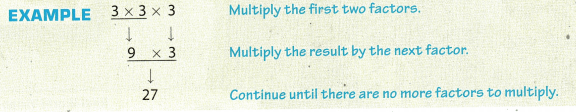Find each product.

Question 5.
8 × 8 × 8centering Find a product of two numbers on the box
8 . 8 = 64
centering Multiply the result by the third number
64 . 8 = 512

Question 6.
5 × 5 × 5centering Multiply the first two numbers.
5 . 5 = 25
centering Multiply the result by the third number
25 . 5 = 125

Question 7.
5.1 × 5.1 × 5.1
Multiply the first two numbersMultiply the result by the third number.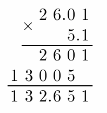132.651 ≈ 132.7

Question 8.
4 × 4 × 4 × 4centering Find a product of two number on the box.
4 . 4 = 16
centering Multiply the result by the third number
16 . 4 = 64

Multiply Fractions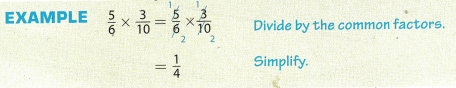Multiply. Write each product in simplest form.

Question 9.
$$\frac{1}{2}$$ × $$\frac{1}{3}$$
Multiply numerator with numerator and denominator with denominator.
$$\frac{1}{2}$$ . $$\frac{1}{3}$$ = $$\frac{1 \cdot 1}{2 \cdot 3}$$
= $$\frac{1}{6}$$

Question 10.
$$\frac{3}{4}$$ × $$\frac{5}{6}$$
Multiply numerator with numerator and denominator with denominator.Question 11.
$$\frac{9}{10}$$ × $$\frac{15}{16}$$
Multiply numerator with numerator and denominator with denominator.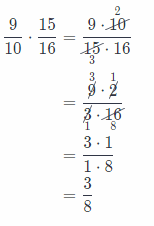Question 12.
$$\frac{2}{5}$$ × $$\frac{5}{12}$$
Multiply numerator with numerator and denominator with denominator.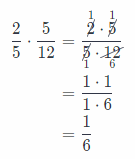Question 13.
$$\frac{4}{7}$$ × $$\frac{3}{16}$$
Multiply numerator with numerator and denominator with denominator.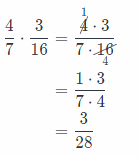Question 14.
$$\frac{5}{12}$$ × $$\frac{3}{20}$$
Multiply numerator with numerator and denominator with denominator.Question 15.
$$\frac{3}{8}$$ × $$\frac{3}{5}$$
Multiply numerator with numerator and denominator with denominator
$$\frac{3}{8}$$ . $$\frac{3}{5}$$ = $$\frac{3 \cdot 3}{8 \cdot 5}$$
= $$\frac{9}{40}$$

Question 16.
$$\frac{5}{9}$$ × $$\frac{7}{10}$$
Multiply numerator with numerator and denominator with denominator.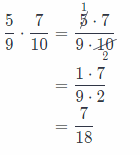Visualize Vocabulary
Use the ✓’ words to complete the graphic. You will put one word in each oval.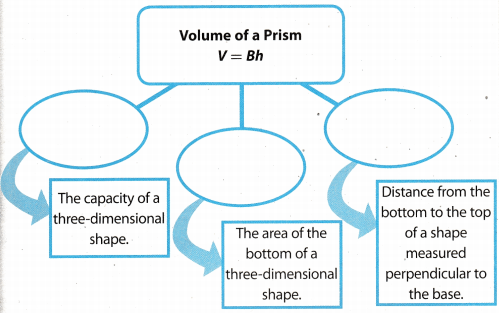Understand Vocabulary

Match the term on the left to the correct expression on the right.